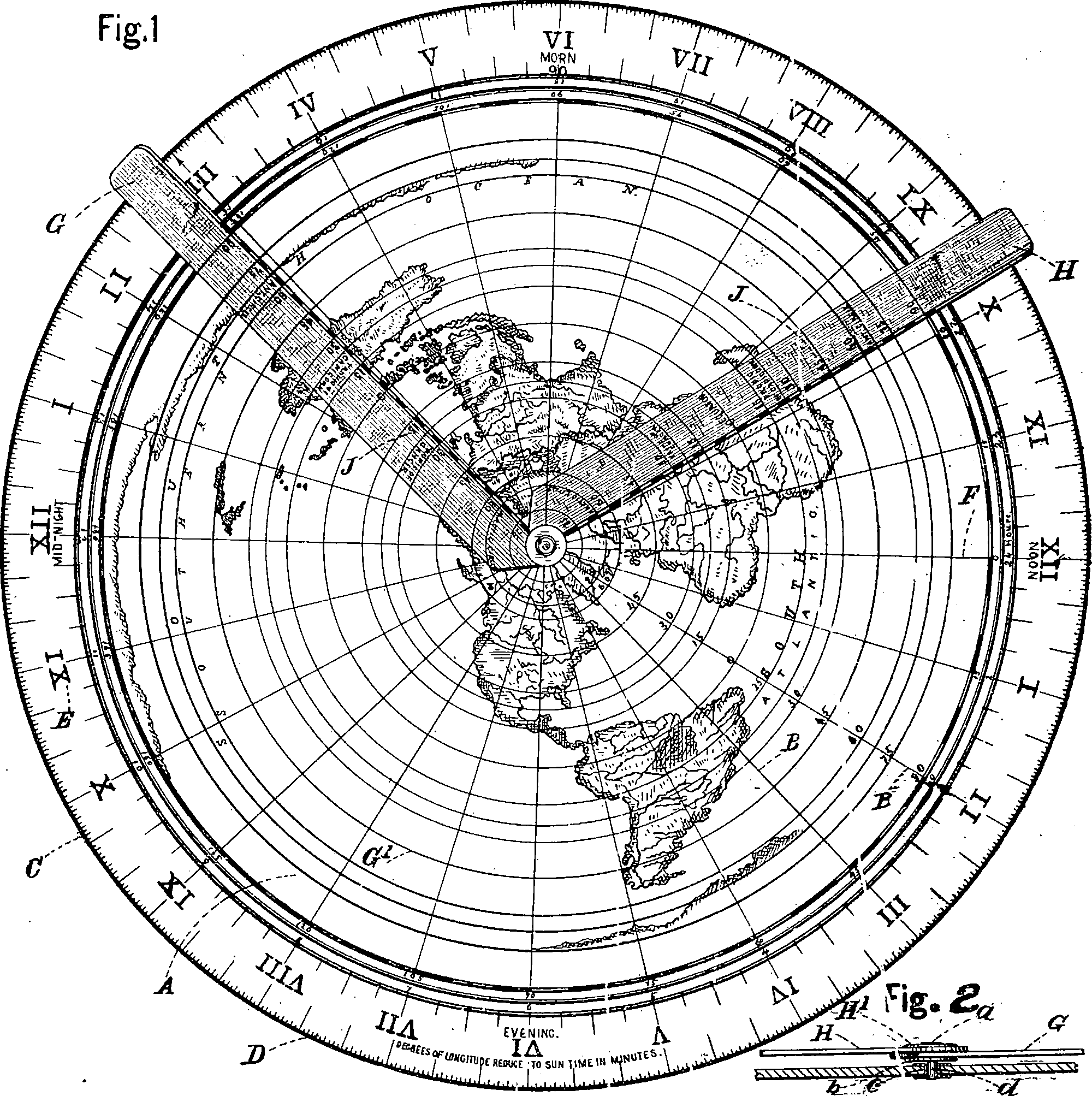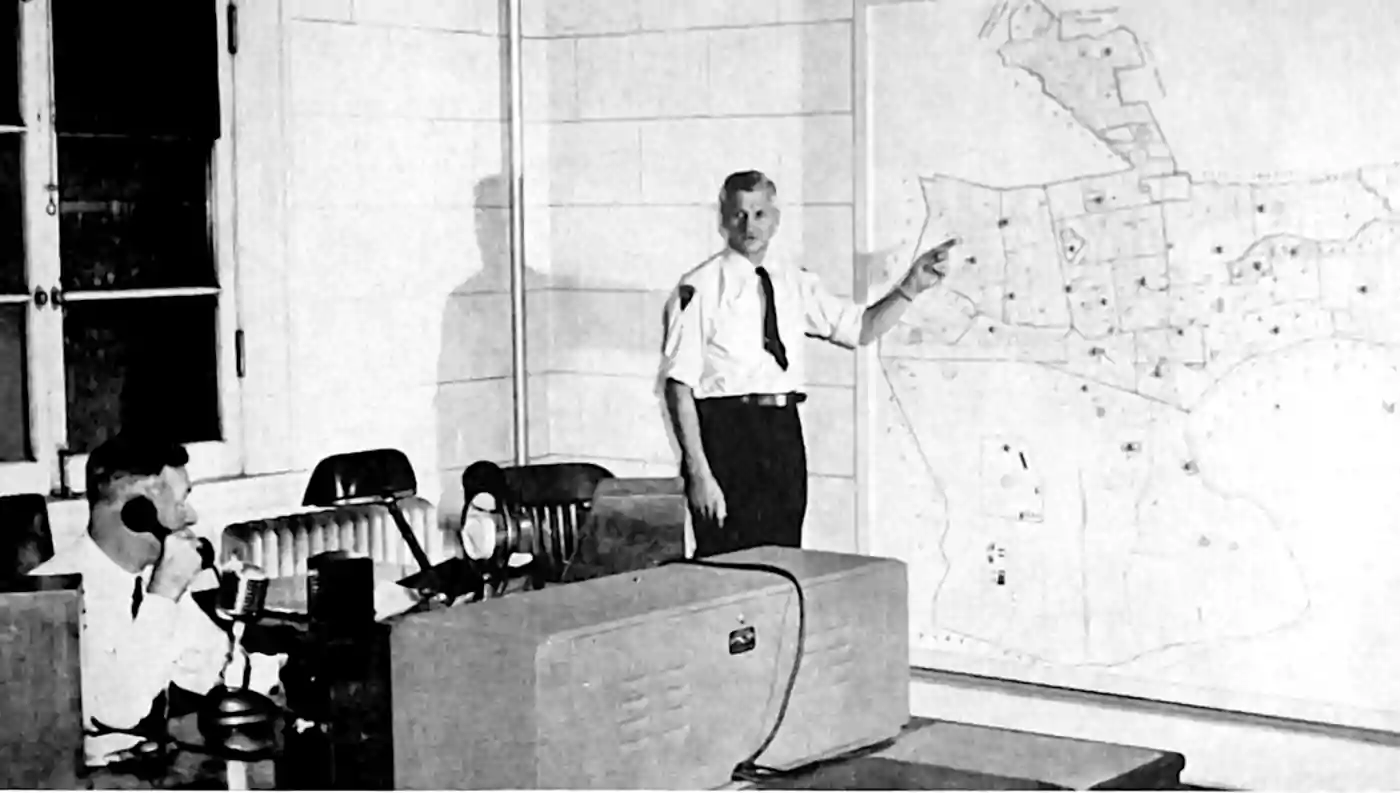# Spatial processes and statistics thereofStatistics on fields with index sets of more than one dimension of support and, frequently, an implicit 2-norm. Sometimes they are also time-indexed. Especially, for processes on a continuous index set with continuous state and undirected interaction. Sometimes over fancy manifolds, although often you can get away with plain old euclidean space, unless you are doing spatial statistics over the entire planet, which turns out to be curved. Lattice models are frequently considered spatial statistics, but more arbitrary graph structures usually get filed under undirected graphical models/random fields. For spatial point processes I will make a new notebook. Often we mean some kind of Gaussian process regression to handle spatial statistics, although the use of these tool in the ML and spatial literatures is weirdly disjoint. There are many other random fields we might also wish to infer that relate to spatial index sets, and these can be taxonomised as I notice their existence. There are lots of interesting problem with statistics on such fields. Consider the illustrative problem of declustering.

I’m curious about how spatial statistics generalise to high-dimensional fields such as fitness landscapes, loss functions, and embedding of network processes in space…

## KrigingThe spatial statistics name for Gaussian process regression. Many complications arise in the spatial context as seen in, e.g. Bayesian inverse problems. Various cunning tricks are needed to make spatial GPs practical

A recent Fixed Rank Kriging paper summarised some approaches

Fixed rank kriging (FRK) is a spatial/spatio-temporal modeling and prediction framework that is scaleable, works well with large datasets, and can deal easily with data that have different spatial supports. FRK hinges on the use of a spatial random effects (SRE) model, in which a spatially correlated mean-zero random process is decomposed using a linear combination of spatial basis functions with random coefficients plus a term that captures the random process’ fine-scale variation. Dimensionality reduction through a relatively small number of basis functions ensures computationally efficient prediction, while the reconstructed spatial process is, in general, non-stationary. The SRE model has a spatial covariance function that is always nonnegative-definite and, because any (possibly non-orthogonal) basis functions can be used, it can be constructed so as to approximate standard families of covariance functions . For a detailed treatment of FRK, see Cressie and Johannesson (2008);Cressie, Shi, and Kang (2010);Nychka et al. (2015).[…]

A few variants of FRK have been developed to date, and the one that comes closest to the present software is LatticeKrig . LatticeKrig implements what we call a LatticeKrig (LTK) model, which is made up of Wendland basis functions (that have compact support) decompos- ing a spatially correlated process. LatticeKrig models use a Markov assumption to construct a precision matrix to describe the dependence between the coefficients of these basis functions. This, in turn, results in efficient computations and the potential use of a large number (> 10,000) of basis functions. LatticeKrig models do not cater for what we term fine-scale-process variation and, instead, the finest scale of the process is limited to the finest resolution of the basis functions used. The package INLA is a general-purpose package for model fitting and prediction. One advantage of INLA is that it contains functionality for fitting Gaussian processes that have covariance functions from the Matérn class (see Lindgren and Rue (2015), for details on the software interface) by approximating a stochastic partial differential equation (SPDE) using a Gaussian Markov random field (GMRF). Specifically, the process is decomposed using basis functions that are triangular ‘tent’ functions, and the coefficients of these basis functions are normally distributed with a sparse precision matrix. Thus, these models, which we term SPDE-GMRF models, share many of the features of LatticeKrig models. A key advantage of INLA over LatticeKrig is that once the spatial or spatio-temporal model is constructed, one has access to all the approximate-inference machinery and likelihood models available within the package.

Kang and Cressie (2011) develop Bayesian FRK; they keep the spatial basis functions fixed and put a prior distribution on K. The predictive-process approach of Banerjee et al. (2008) can also be seen as a type of Bayesian FRK, where the basis functions are constructed from the postulated covariance function of the spatial random effects and hence depend on parameters (see Katzfuss and Hammerling (2017), for an equivalence argument). An R package that implements predictive processes is spBayes . It allows for multivariate spatial or spatio-temporal processes, and Bayesian inference is carried out using Markov chain Monte Carlo (MCMC), thus allowing for a variety of likelihood models.

Spatial processes are unlikely to be truly Gaussian; we often justify the approximation with a Gaussian process by Laplace approximation.

## Spatial point processesA particular sub-case combining point processes with spatial statistics, now with its own notebook

## Tools

All recommendations made to me and passed on here are offered unreviewed and unendorsed.

### R

Spatial statistics in R is diverse enough to need its own notebook. Check there.

### Python

Spatial statistics in Python is diverse enough to need its own notebook. Check there.

### QGIS

QGIS is a free and open-source cross-platform desktop geographic information system (GIS) application that supports viewing, editing, and analysis of geospatial data.

Notably it support embedded python. It seems to be a GIS system, in that it places geography first and statistics second.

### No comments yet. Why not leave one?

GitHub-flavored Markdown & a sane subset of HTML is supported.